Question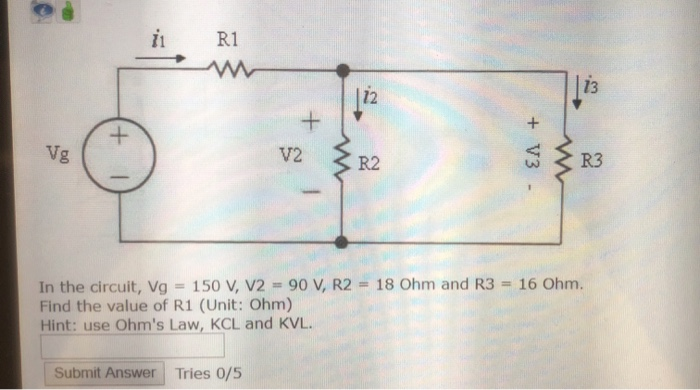We need at least 10 more requests to produce the answer.

0 / 10 have requested this problem solution

The more requests, the faster the answer.

All students who have requested the answer will be notified once they are available.

#### Earn Coins

Coins can be redeemed for fabulous gifts.

Similar Homework Help Questions
• ### R1 R2 R4 Is R3 Given that Vs is 18 V, Is is 6 A, R1...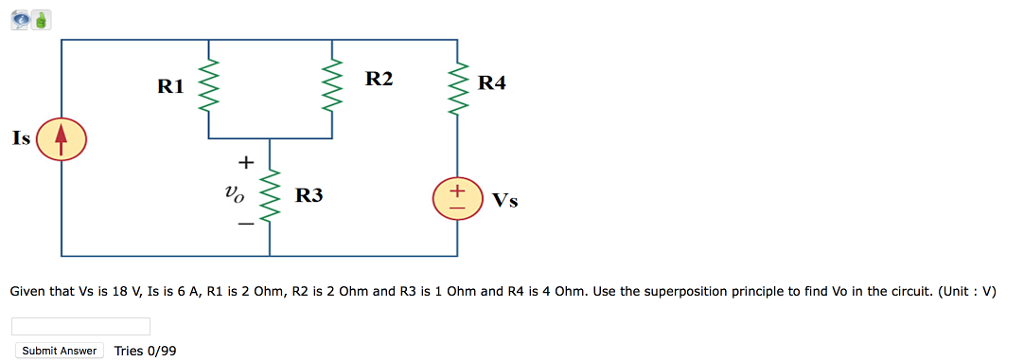R1 R2 R4 Is R3 Given that Vs is 18 V, Is is 6 A, R1 is 2 Ohm, R2 is 2 Ohm and R3 is 1 Ohm and R4 is 4 Ohm. Use the superposition principle to find Vo in the circuit. (Unit V) Submit Answer Tries 0/99

• ### pls help R1 R3 R2 w w V14 V3+ V2+ Consider the circuit shown below, where...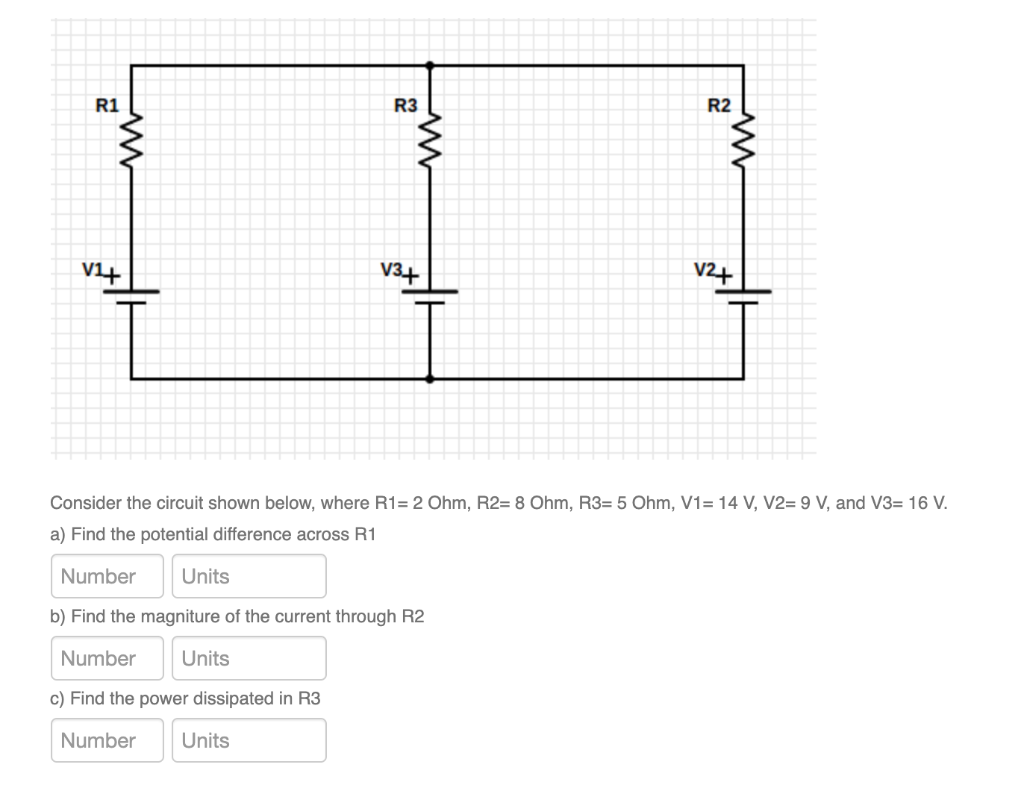pls help R1 R3 R2 w w V14 V3+ V2+ Consider the circuit shown below, where R1= 2 Ohm, R2= 8 Ohm, R3= 5 Ohm, V1= 14 V, V2= 9 V, and V3= 16 V. a) Find the potential difference across R1 Number Units b) Find the magniture of the current through R2 Number Units c) Find the power dissipated in R3 Number Units

• ### In the circuit, V1=129 V, V2=72 V, I0=2 A, R1=7 Ohm, R2=46 Ohm, R3=19 Ohm, R4=19...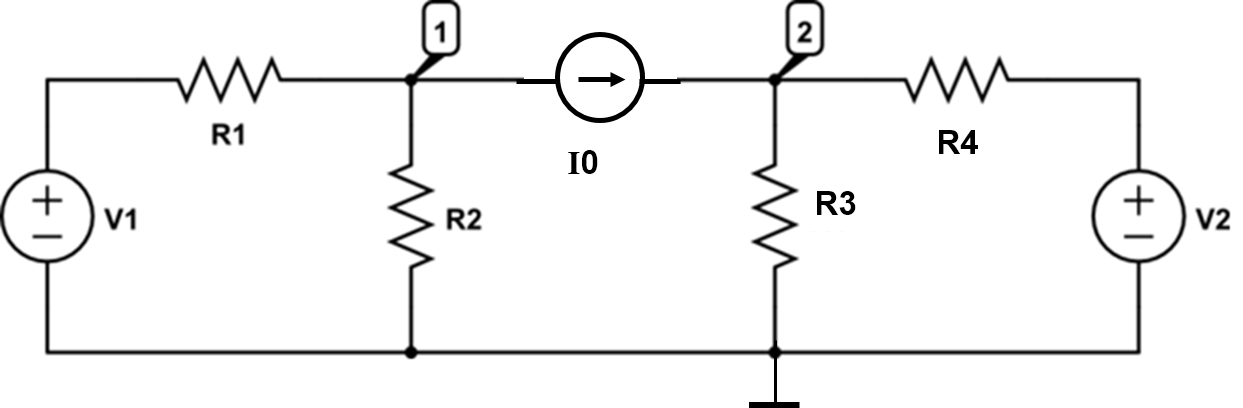In the circuit, V1=129 V, V2=72 V, I0=2 A, R1=7 Ohm, R2=46 Ohm, R3=19 Ohm, R4=19 Ohm. Solve the circuit using nodal analysis and answer the questions below. Find the current through R1 flowing in the direction from left to right. (Unit: A) Find the current through R2 flowing downwards. (Unit: A) Find the current through R3 flowing downwards. (Unit: A) Find the current thrrough R4 flowing from left to right. (Unit: A) R1 R4

• ### R2 R3 R1 C1 C2 S2 51 In the circuit, the signal frequency f = 1.2 MHz, R1=9 Ohm, R2 = 8 Ohm, R3 =...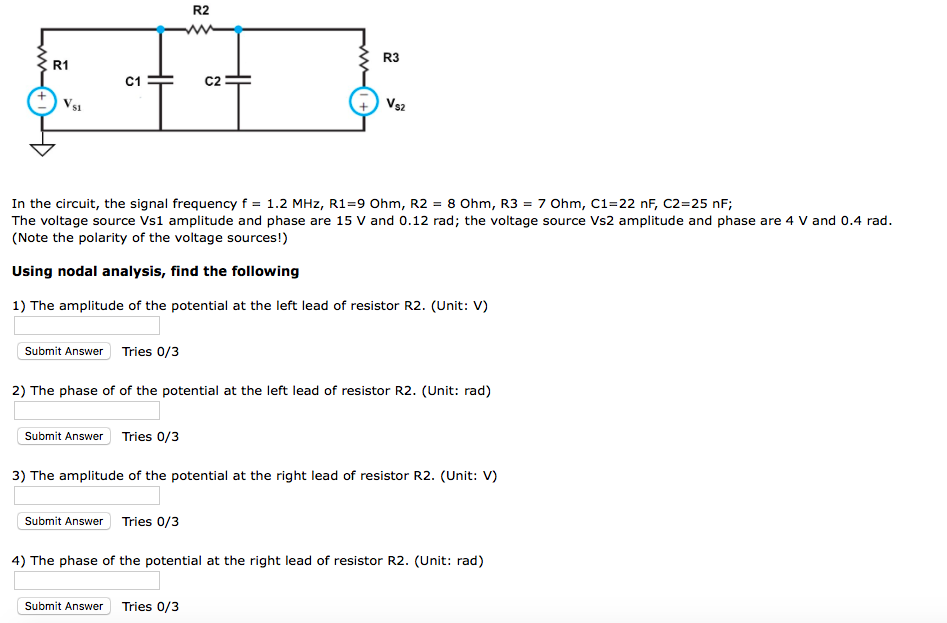PLEASE HELP IMMEDIATELY R2 R3 R1 C1 C2 S2 51 In the circuit, the signal frequency f = 1.2 MHz, R1=9 Ohm, R2 = 8 Ohm, R3 = 7 Ohm, C1-22 nF, C2=25 nF; The voltage source Vs1 amplitude and phase are 15 V and 0.12 rad; the voltage source Vs2 amplitude and phase are 4 V and 0.4 rad. (Note the polarity of the voltage sources!) Using nodal analysis, find the following 1) The amplitude of the potential at...

• ### For the circuit shown in the figure, If R1=512, R2=10N, R3=3N, V1= V1}V, V2=5V and V3=7V....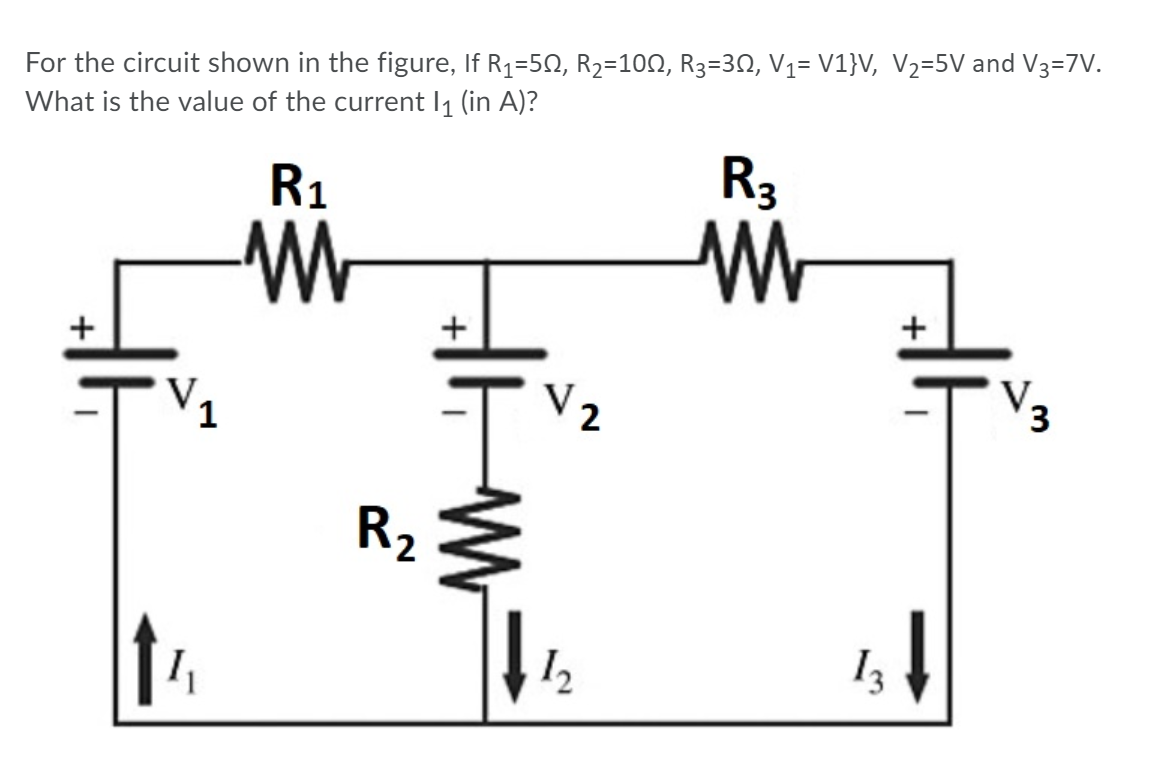For the circuit shown in the figure, If R1=512, R2=10N, R3=3N, V1= V1}V, V2=5V and V3=7V. What is the value of the current 11 (in A)? R1 -W R3 M + + V2 V. 3 R2 \$ 12 13

• ### vi Ri ii V3 R4 V5 Rs jis R7 In the circuit, Vg = 126 V,...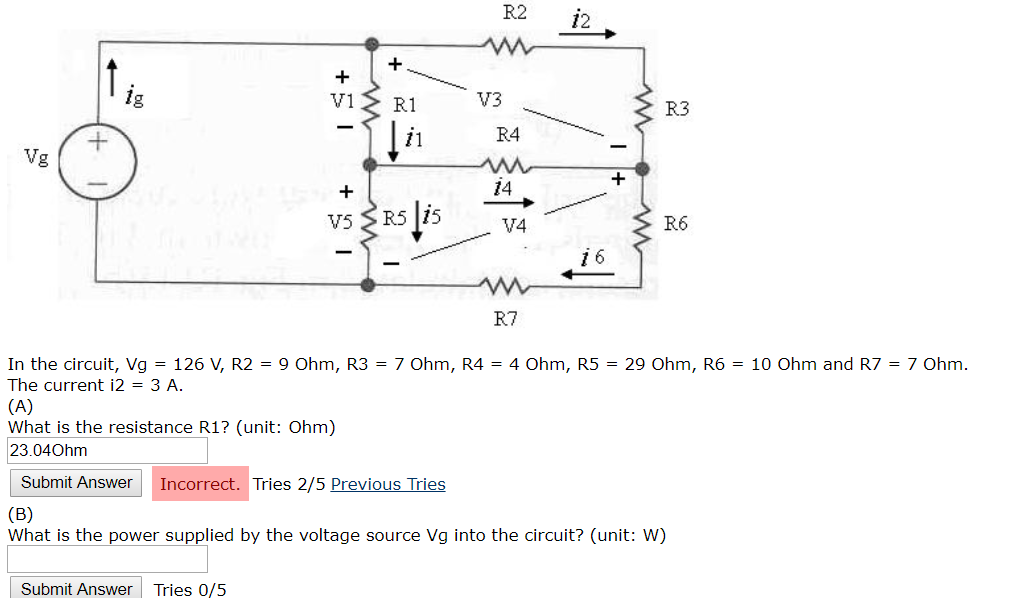vi Ri ii V3 R4 V5 Rs jis R7 In the circuit, Vg = 126 V, R2 = 9 Ohm, R3 = 7 Ohm, R4 = 4 Ohm, R5 = 29 Ohm, R6 = 10 Ohm and R7 = 7 Ohm. The current i2 = 3 A. (A) What is the resistance R1? (unit: Ohm) 23.04Ohm Submit Answer Incorrect. Tries 2/5 Previous Tries (B) What is the power supplied by the voltage source Vg into the circuit? (unit: W) Submit...

• ### R1 12 R2 13 R3 V = 10 V, R1 = 50, R2 = 100, R3...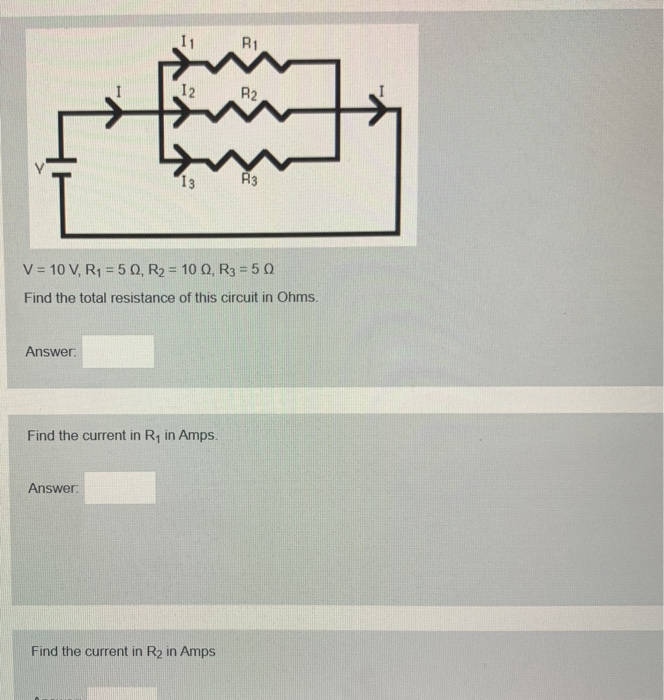R1 12 R2 13 R3 V = 10 V, R1 = 50, R2 = 100, R3 = 50 Find the total resistance of this circuit in Ohms. Answer: Find the current in Ry in Amps Answer: Find the current in R2 in Amps Find the current in R3 in Amps Answer: Find the voltage drop in Volts across R1. Answer: Find the voltage drop in Volts across R2. Answer:

• ### In the circuit shown, R1 = 7 ohm, R2 = 11 ohm, R3 = 9ohm, V1...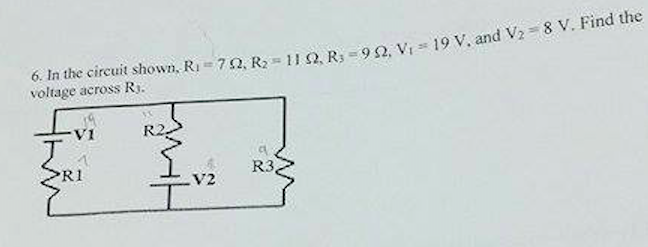In the circuit shown, R1 = 7 ohm, R2 = 11 ohm, R3 = 9ohm, V1 = 19 V, and V2 = 8V. Find the voltage across R3.

• ### In the circuit, R1=50 Ohm, R2 = 36 Ohm, the reactance of the inductor L1 is...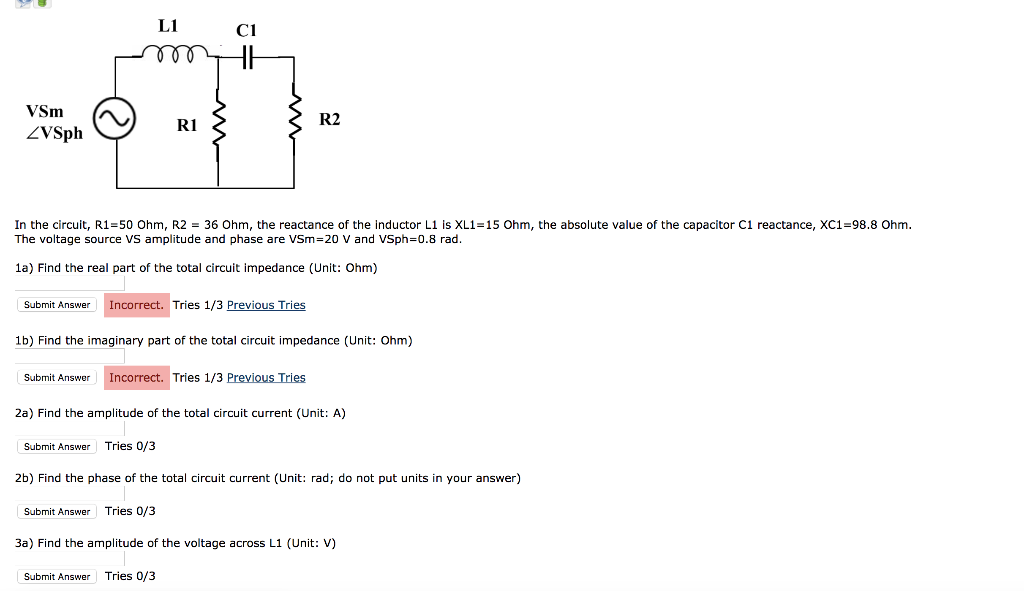In the circuit, R1=50 Ohm, R2 = 36 Ohm, the reactance of the inductor L1 is XL1=15 Ohm, the absolute value of the capacitor C1 reactance, XC1=98.8 Ohm. The voltage source VS amplitude and phase are VSm=20 V and VSph=0.8 rad. L1C1 om R2 VSm ZVSph ~ R13 R2 In the circuit, R1=50 Ohm, R2 = 36 Ohm, the reactance of the inductor Li is XL1=15 Ohm, the absolute value of the capacitor C1 reactance, XC1=98.8 Ohm. The voltage source...

• ### PI : For circuit below v-20 V and R,-8 Ohm and R2-2 Ohm. Calculate voltage and...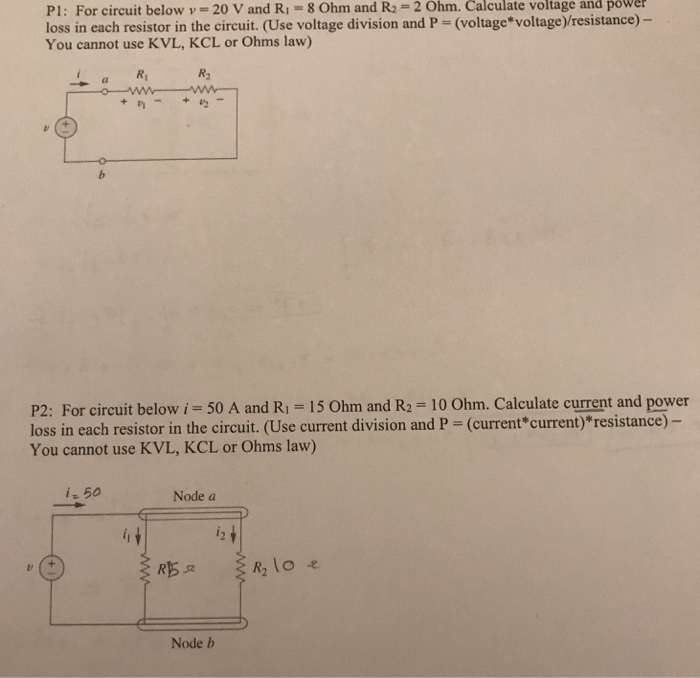PI : For circuit below v-20 V and R,-8 Ohm and R2-2 Ohm. Calculate voltage and power loss in each resistor in the circuit. (Use voltage division and P = (voltage*voltage) resistance)- You cannot use KVL, KCL or Ohms law) Ri R2 P2: For circuit below i = 50 A and R1 = 15 Ohm and R2 = 10 Ohm. Calculate current and power loss in each resistor in the circuit. (Use current division and P - (current*current) resistance) You...